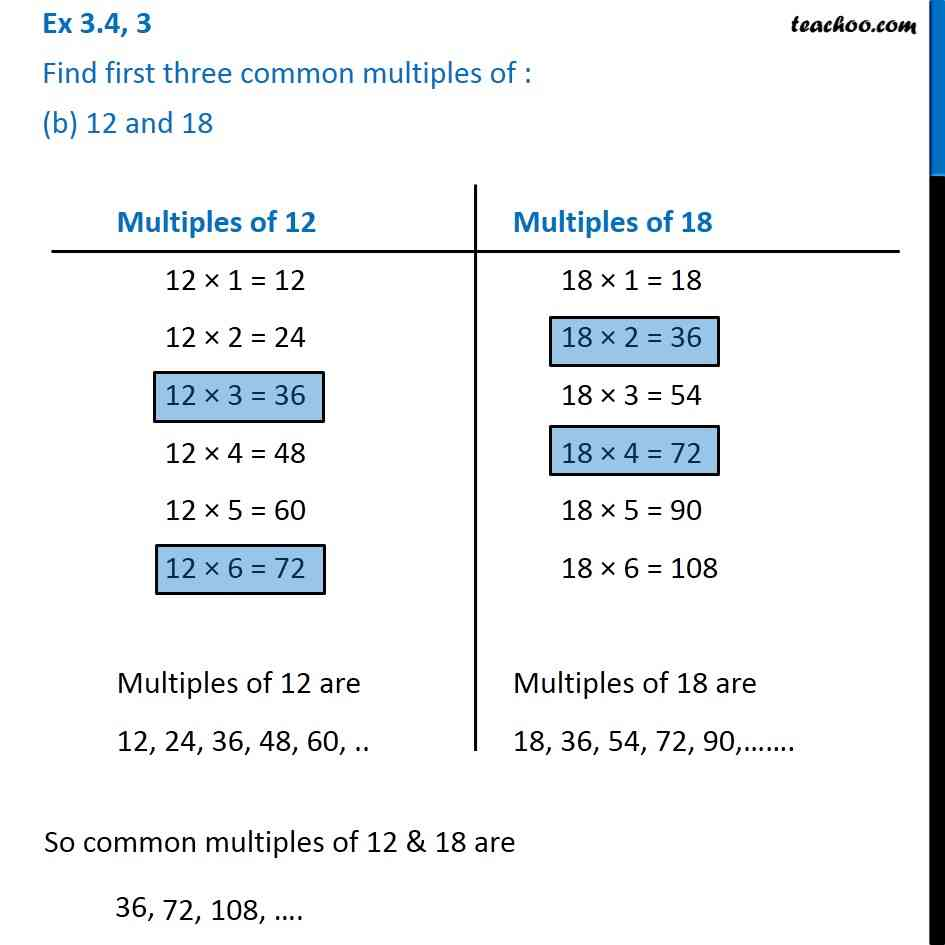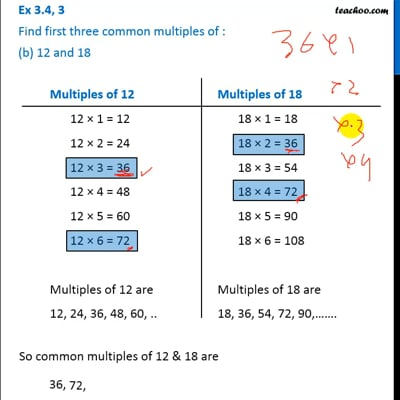Ex 3.4

Chapter 3 Class 6 Playing with Numbers
Serial order wiseThis video is only available for Teachoo black users

Introducing your new favourite teacher - Teachoo Black, at only ₹83 per month

### Transcript

Ex 3.4, 3 Find first three common multiples of : (b) 12 and 18Multiples of 12 12 × 1 = 12 12 × 2 = 24 12 × 3 = 36 12 × 4 = 48 12 × 5 = 60 12 × 6 = 72 Multiples of 12 are 12, 24, 36, 48, 60, .. Multiples of 18 18 × 1 = 18 18 × 2 = 36 18 × 3 = 54 18 × 4 = 72 18 × 5 = 90 18 × 6 = 108 Multiples of 18 are 18, 36, 54, 72, 90,……. So common multiples of 12 & 18 are 36,